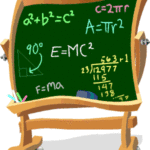# How to study Mathematics properly, What is the Best wayMathematics is a subject which once understood is never forgotten but if not studied well is never interesting and to pass the subject is a herculean task. But, it is never so.

The numero phobia should be thrown away, and a proper scientific approach should be made. And yes a consistency of regular practice is also needed.

• Studying all the important dates, facts and lengthy answers just before a history examination will pass us, but it is not the cashew in maths. Studying maths a day before exam will only make matters worse. So studies should be done for a long time before examinations.
• There are many books in the market and lots of suggestions from everyone. However, only that book should be chosen with which we can understand properly. In addition, a good textbook can never be substituted by anything.
• Before starting the exercise, the theory part and the solved examples should be reviewed very carefully. The text part gives a basic idea of what the exercise is all about. The solved examples are to be reviewed then solved again. Once these two activities are over the exercise should be started.
• The restrictions of each formula should be learnt. For example, to use the quadratic formula, the equation must be in the quadratic form first. These applications must be understood well. Formulas should never be learnt by heart. It is very difficult to learn the formula for integration by parts. These can only be remembered by regular practice.
• It should be remembered that mathematics is cumulative. Everything presently being studied is based on past studies. Performing calculus is very difficult without having the basic knowledge of trigonometry and algebra. So, every section should be taken care of and managed well.
• While solving a sum there are some basic steps:
1. The sum should be read very carefully. The concept should be understood well. What is actually given and what is being asked should be known.
2. All the information given should be noted down, and special attention should be paid while writing numbers.
3. Using proper formulas, the sum must be solved. If answer does not come then the question must be reviewed again.
• There are two approaches to a sum, one is conceptual approach and the other is a numerical approach. The conceptual approach gives a correct formula and opinion to solve a sum and numerical approach hives a correct answer. So, both these approaches need to be looked after for getting the right answer.

Now, studying mathematics is a child play and each sum should be enjoyed.

Topic:  Other